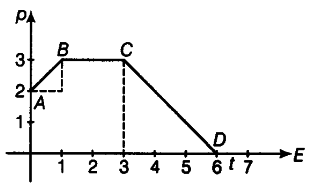# Figure shows versus graph far a particle moving along x-axis

Figure shows versus graph far a particle moving along x-axis. In which region, farce on the particle is large.
Why ?Net force is given by \$F_{ net }\$ = dp / dt
Also, rate of change of momentum = slope of graph.
As from graph,\$slope_{ AB }\$ = \$slope_{CD}\$
And slope (BC)= slope (DE)=0 W
So, force acting on the particle is equal in regions AB and CD and in regions BC and DE (which is zero).